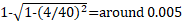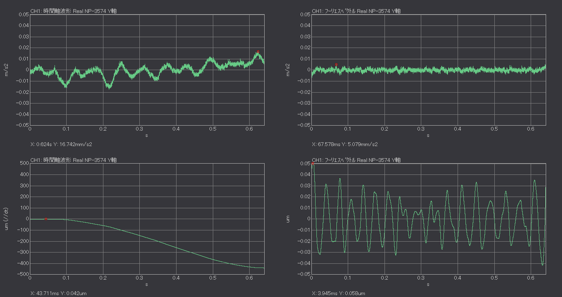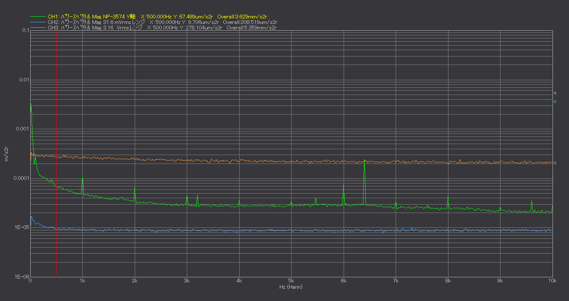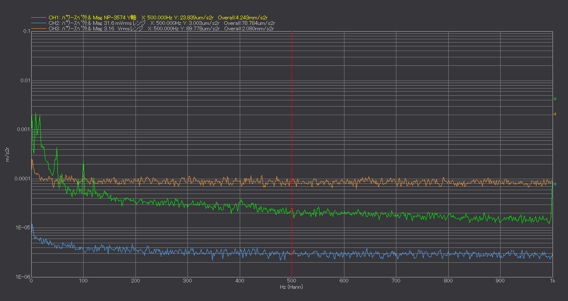One of the most frequently asked questions about accelerometers is related to the minimum measurable acceleration, vibration velocity, and displacement. If there is no background vibration or electrical noise, and the self-noise of the analyzer is sufficiently small, the minimum measureable acceleration is decided according to the detector noise of the accelerometer (in the case of a charge output type accelerometer, the input conversion noise of the charge amplifier)

•Detector noise of Accelerometer

The detector noise of the built-in preamplifier accelerometer is indicated in the product specification. The detector noise of tri-axial accelerometer NP-3574 and NP-3578N20 is 0.004 m/s2 (rms) or less. It means the equivalent to 4 mm/s2 (rms) of voltage signal is output.

The error is about 4 mm/s2 when measuring the time waveform of acceleration or measuring the maximum value of the time waveform. If the measurement result is 40 mm/s2, the error will be ± 4 mm/s2 (±10%). If the measurement result is 400 mm/s2, the error will be ± 4 mm/s2 (±1%). If a measurement accuracy of around 10% is acceptable, the minimum measureable acceleration is 40 mm/s2, which is 10 times the detector noise.

When the arithmetic mean of the rms value or power spectrum is measured, the effect of detector noise becomes less since the calculation is performed by the square value of acceleration. If the measurement result is 40 mm/s2, the error is, 0.5%. If the magnitude of acceleration is 10 times as the detector noise, the sufficient accuracy will be obtained.

As for vibration velocity, and displacement, as the relationship between acceleration value and vibration velocity/ displacement depends on the frequency distribution of vibration, they are not able to be estimated by the detector noise of accelerometer. They should be verified to measure the vibration of target object.

Charge output type accelerometer and the input conversion noise of charge amplifier

If using the charge output type accelerometer, the noise depends on the input conversion noise of the charge amplifier. The input conversion noise of the charge amplifier, CH-1200A is 0.05 pC (rms) or less. When CH-1200A is combined with NP-2106, 0.05 pC (rms) is equivalent to 14.3 m/s2 as the sensitivity of NP-2106 is around 0.035 pC/ (m/s2). If the minimum measurable acceleration is 10 times of the detector noise, the minimum acceleration measured by NP-2106 is 14.3 m/s2. NP-2106 is not suitable for the small acceleration but for the large one for the drop/ impact test.

•Time waveform of detector noise

The time waveform of the noise measured by placing Accelerometer NP-3574 on the shelf is shown in Figure 1. This signal includes the detector noise as well as background vibration or electrical noise. The sensitivity of NP-3574 is 10 mV/ (ms2), the detector noise is 0.004 m/s2 or less. It is measured at 10 kHz of frequency range, 31.6 mVrms of voltage range.

The time waveform of the noise signal output from accelerometer is shown in the upper left of Figure 1. It includes the low-frequency of background vibration and electrical noise, and its peak value is 16.742 m/s2.  The displacement waveform by double integration of the upper left waveform is shown in the lower left of Figure 1. The waveform has a downward-sloping waveform due to the low-frequency component of the original signal, and the minimum value is -440 μm. This is not the actual displacement waveform but the incorrect waveform obtained by numerical integration of a signal containing low frequency noise.

The time waveform of acceleration with components below 25 Hz cut using inverse FFT function is shown in the upper right of Figure 1. The peak value is 5.079 m/s2. The displacement waveform obtained by double integration to cut components below 25 Hz is shown in the lower right of Figure 1, its peak value is 0.058 μm.

The actual measurements are affected by background vibration and electrical noise in addition to the detector noise of the accelerometer. Therefore, it is necessary to measure the real noise signal and check the measureable acceleration and displacement values.Fig.1 Time waveform of detector noise
Upper left: Acceleration waveform, Upper right: Band limiting acceleration waveform
Lower left: Double integration waveform, Lower right: Band limiting double integration waveform

Power spectrum of Detector noise (1)

The power spectrum of the noise measured by placing Accelerometer NP-3574 on the shelf is shown in Figure 2 (green). This signal includes the detector noise as well as background vibration or electrical noise. The sensitivity of NP-3574 is 10 mV/(ms2), the detector noise is 0.004 m/s2 or less. The arithmetic mean is performed by 10 kHz of frequency range, sampling points of 2048 points, 31.6 mVrms of voltage range.

The self-noise of DS-3000 is shown in orange, Figure 2, when the voltage range is set as 1 Vrms. The small vibration cannot be measured if the voltage range remains 1 Vrms since it is larger than NP-3574’s noise. If the vibration of a target object to be measured is small, input over should not occur even if the voltage range is lowered. Please set the voltage range as small as possible without causing input over.

Each frequency component value is quite smaller than the self-noise of detector (4 mm/s2). It is necessary to measure real background vibration, electrical noise at site. In addition, the measurement can be done if the vibration to be measured is sufficiently larger than those noises, otherwise, it is necessary to determine other measurement method that is not much affected by background vibration, electrical noise.

If single integration or double integration is performed against the noise power spectrum, the noise spectrum converted to vibration velocity or displacement can be calculated by comparing the vibration velocity and displacement values you want to measure, or the actual vibration velocity and displacement measurement results of the target, you can determine whether measurement can be done.Fig.2 The noise power spectrum (10 kHz range)
Green: NP-3574,Blue: DS-3000 (31.6 mVrms range), Orange: DS-3000 (1 Vrms range)

Power spectrum of Detector noise (2)

The power spectrum for the noise of NP-3574 at 1 kHz frequency range and the self-noise of DS-3000 (31.6 mVrms range, 1 Vrms range) are shown in Figure 3. NP-3574 noise includes the detector noise as well as background vibration or electrical noise.

The components below 50 Hz or 20 Hz are remarkable as the frequency range is lowered. Although the place where this measurement is performed is a general office, the low frequency components below 20 Hz and the frequency components of 50 Hz and 100 Hz generated from commercial power are still observed. The measurement can be done if the vibration to be measured is sufficiently larger than those, otherwise, it is necessary to determine other measurement method that is not much affected by background vibration, electrical noise.

If single integration or double integration is performed against the noise power spectrum, the noise spectrum converted to vibration velocity or displacement can be calculated by comparing the vibration velocity and displacement values you want to measure, or the actual vibration velocity and displacement measurement results of the target, you can determine whether measurement can be done.Fig.3 The noise power spectrum (1 kHz range)
Green: NP-3574, Blue: DS-3000 (31.6 mVrms range), Orange: DS-3000 (1 Vrms range)

Summary

In this column, we explain about the minimum measurable acceleration, vibration velocity, and displacement.

The minimum acceleration to be measured by the accelerometer is obtained by its specification (detector noise). However, it is necessary to measure the noise at the measurement site and check how much acceleration can be measured since it is affected by background vibration and electrical noise at the site.

Since the relationship between the acceleration and vibration velocity/ displacement depends on the frequency distribution of vibration, the minimum vibration velocity/ displacement to be measured is not obtained by the specification of detector. It is necessary to check how much value can be measured upon measuring the vibration level and frequency distribution of the measurement object.

(Y.K)